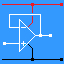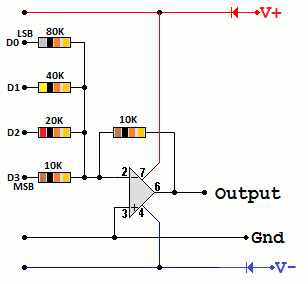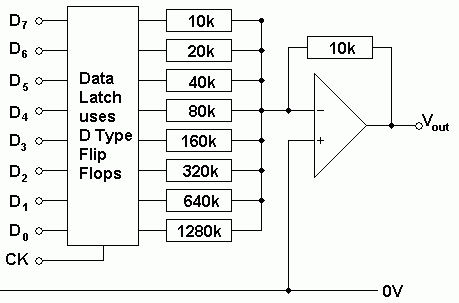RANDOM PAGE

SITE SEARCH

LOG
IN

HELP

# D to A Converter-Summing

This is the AQA version closing after June 2019. Visit the the version for Eduqas instead.

## Summing Amplifier Converter

• The input resistors have values that are in multiples of 2.
• For example 10k, 20k, 40k, 80k, 160k, 320k etc.
• This gives input gains of one, half, quarter, eighth, sixteenth, etc.
• Binary 0 or 1 values on the input are added together but the gain on each input gives the correct weighting to each bit.
• Binary 0 = 0V
• Binary 1 = 5VIn this diagram if the binary input is 0101, using the summing amplifier formula ...

Vout = -10( 0 / 10 + 5 / 20 + 0 / 40 + 5 / 80 ) = 250 / 80 = -3.125 Volts

## Resolution

Resolution is the size of the voltage steps as the digital data is incremented (add one) or decremented (subtract one).

0.625 Volts is the size of the smallest step. This is the resolution of the DAC.

## Digital Ramp

If the DAC above is driven with a signal from a binary up counter increasing from 0 to 15, the output voltage will decrease in steps of 0.625 Volts.

This gives an output like this ...## 8 Bit Latch and Summing DACHere is a diagram showing an 8 bit DAC with a data latch. On the rising edge of the clock pulse, the data is latched (stored in the latch). The latch is needed if the data input not always available. When the input is valid, the rising edge of the clock causes the latch to store the data.

For example, this circuit might be connected to the data bus of a computer. Most of the time the bus is carrying data that is nothing to do with the DAC output. If the DAC is memory mapped, an address decoder would be used to trigger the clock in response to a particular address.

reviseOmatic V3     Contacts, ©, Cookies, Data Protection and Disclaimers Hosted at linode.com, London